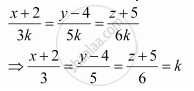Share

# Find the Cartesian Equation of the Line Which Passes Through the Point (−2, 4, −5) and Parallel to the Line Given by (X+3)/3 = (Y-4)/5 = (Z+8)/6 - CBSE (Commerce) Class 12 - Mathematics

#### Question

Find the Cartesian equation of the line which passes through the point (−2, 4, −5) and parallel to the line given by (x+3)/3 = (y-4)/5 = ("z"+8)/6

#### Solution

It is given that the line passes through the point (−2, 4, −5) and is parallel to

(x+3)/3 = (y-4)/5 = (z+8)/6

The direction ratios of the line,

(x+3)/3 = (y-4)/5 = (z+8)/6 are 3, 5, and 6.

The required line is parallel to (x+3)/3 = (y-4)/5 = (z+8)/6

Therefore, its direction ratios are 3k, 5k, and 6k, where k ≠ 0

It is known that the equation of the line through the point (x1y1z1) and with direction ratios, abc, is given by (x-x_1)/a = (y-y_1)/b = (z-z_1)/c

Therefore the equation of the required line isIs there an error in this question or solution?

#### APPEARS IN

NCERT Solution for Mathematics Textbook for Class 12 (2018 to Current)
Chapter 11: Three Dimensional Geometry
Q: 6 | Page no. 477

#### Video TutorialsVIEW ALL 

Solution Find the Cartesian Equation of the Line Which Passes Through the Point (−2, 4, −5) and Parallel to the Line Given by (X+3)/3 = (Y-4)/5 = (Z+8)/6 Concept: Equation of a Line in Space.
S